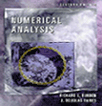# Numerical Analysis

## Programs Supporting Algorithms

Applets and Wed design by Richard Nunoo, book by Dr. Richard Burden and Dr. D. Faires.

These programs based on the Algorithms in the text give correct results for the examples and exercises in the text, but no attempt was made to write a general-purpose professional software. Specially, the algorithms are not always in the form that leads to most efficient program in terms of time and storage requirements. Expression capturing is powered by JEP. You need Java plug-in to run applets. You can also download Netscape 7.1 which install Java plug-in automatically.

CHAPTER 1 CHAPTER 2 CHAPTER 3 CHAPTER 4 CHAPTER 5 CHAPTER 6
Algorithm 2.1 Algorithm 3.1 Algorithm 4.1 Algorithm 5.1 Algorithm 6.1
Algorithm 2.2 Algorithm 3.2 Algorithm 4.2 Algorithm 5.2 Algorithm 6.2
Algorithm 2.3 Algorithm 3.3 Algorithm 4.3 Algorithm 5.3 Algorithm 6.3
Algorithm 2.4 Algorithm 3.4 Algorithm 4.4 Algorithm 5.4 Algorithm 6.4
Algorithm 2.5 Algorithm 3.5 Algorithm 4.5 Algorithm 5.5 Algorithm 6.5
Algorithm 2.6 Algorithm 3.6 Algorithm 4.6 Algorithm 5.6 Algorithm 6.6
Algorithm 2.7     Algorithm 5.7 Algorithm 6.7
Algorithm 2.8     Algorithm 5.8

CHAPTER 7 CHAPTER 8 CHAPTER 9 CHAPTER 10 CHAPTER 11 CHAPTER 12
Algorithm 7.1 Algorithm 8.1 Algorithm 9.1 Algorithm 10.1 Algorithm 11.1 Algorithm 12.1
Algorithm 7.2 Algorithm 8.2 Algorithm 9.2 Algorithm 10.2 Algorithm 11.2 Algorithm 12.2
Algorithm 7.3 Algorithm 8.3 Algorithm 9.3 Algorithm 10.3 Algorithm 11.3 Algorithm 12.3
Algorithm 7.4   Algorithm 9.4 Algorithm 10.4 Algorithm 11.4 Algorithm 12.4
Algorithm 7.5   Algorithm 9.5   Algorithm 11.5 Algorithm 12.5
Algorithm 9.6   Algorithm 11.6